# Alisamento exemplo-2

• Nota de Estudos
• Rever Tópicos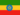Henock A. 0 0 done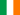Rachel N. 0 0 Smoothing - example 1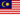Tin Myo H. 0 0 why do we need to do smoothing in trends analysis please?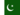Hanif Ur R. 0 0 nice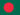Jahidul I. 0 0 Weighted average form The forecast at time t+1 is equal to a weighted average between the most recent observation yt and the most recent forecast y^t|t−1, y^t+1|t=αyt+(1−α)y^t|t−1 for t=1,…,T, where 0≤α≤1 is the smoothing parameter. The process has to start somewhere, so we let the first forecast of y1 be denoted by ℓ0. Then y^2|1y^3|2y^4|3⋮y^T+1|T=αy1+(1−α)ℓ0=αy2+(1−α)y^2|1=αy3+(1−α)y^3|2=αyT+(1−α)y^T|T−1 Then substituting each equation into the following equation, we obtain y^3|2y^4|3=αy2+(1−α)[αy1+(1−α)ℓ0]=αy2+α(1−α)y1+(1−α)2ℓ0=αy3+(1−α)[αy2+α(1−α)y1+(1−α)2ℓ0]=αy3+α(1−α)y2+α(1−α)2y1+(1−α)3ℓ0 ⋮ y^T+1|T=∑j=0T−1α(1−α)jyT−j+(1−α)Tℓ0.(7.2) So the weighted average form leads to the same forecast equatioJahidul I. 0 0 Describe the Smoothing Time Series ?
• Text Version### Você recebeu uma nova notificação

Clique aqui para visualizar todos eles